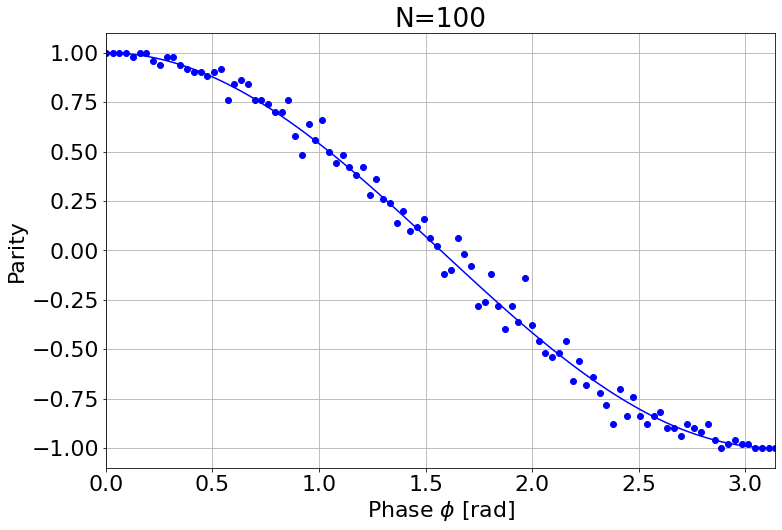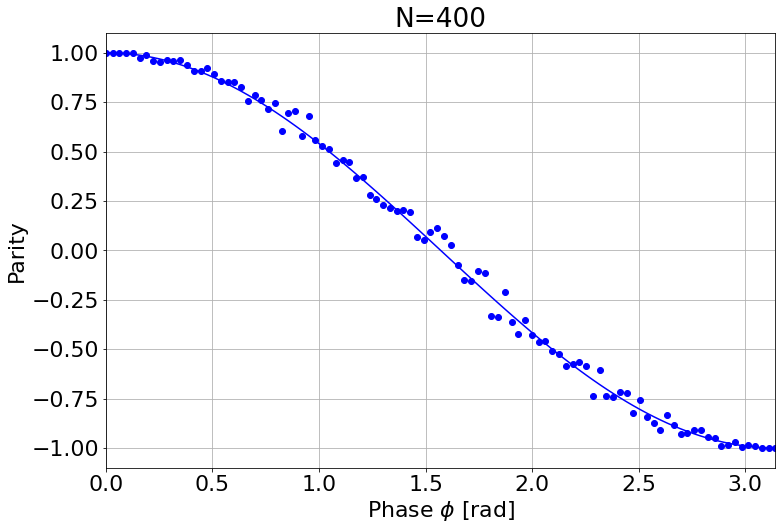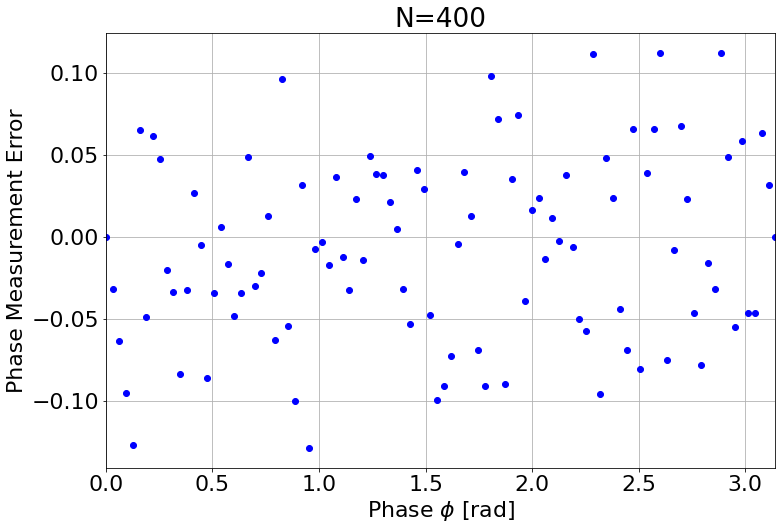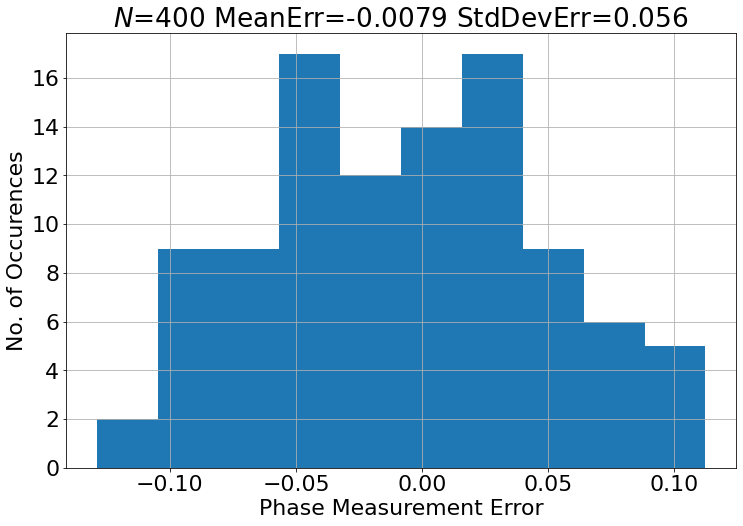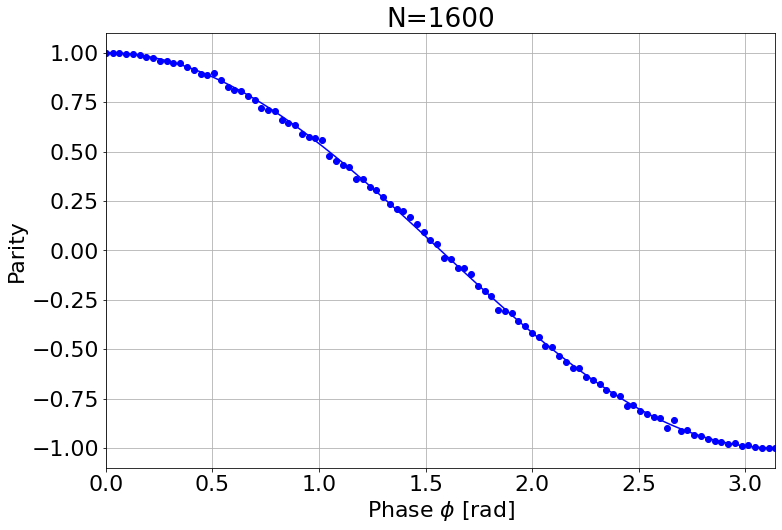# Quantum Phase Measurment (Clock Synchronization)¶

This experiments is based on key scientific papers, which are:

• Komar, Peter, et al. "A quantum network of clocks." Nature Physics 10.8 (2014): 582-587.
• Giovannetti, Vittorio, Seth Lloyd, and Lorenzo Maccone. "Quantum-enhanced positioning and clock synchronization." Nature 412.6845 (2001): 417-419.
• Bollinger, John J., et al. "Optimal frequency measurements with maximally correlated states." Physical Review A 54.6 (1996): R4649.
• Leibfried, Dietrich, et al. "Toward Heisenberg-limited spectroscopy with multiparticle entangled states." Science 304.5676 (2004): 1476-1478.
• Kimble, H. Jeff. "The quantum internet." Nature 453.7198 (2008): 1023-1030.

A clock in the most simple sense is a resonator with a well-defined frequency $\omega_0$. The process of time-keeping is nothing more then the frequent interrogation of the clocks phase $\phi$, which ticks with a rate of $\phi=\omega_0t$ and thus $t=\phi/\omega_0$. In the current SI system $\omega_0$ is defined by the frequency of the unperturbed ground-state hyperfine transition of the caesium 133 atom, which is defined to be $9,192,631,770 Hz$. A the trasition frequency is know (defined, actually), the process of time-keeping requires us to frequently interrogate the phase with the higest possible precision. Assuming that the iterrogation delay is fixed, we a timing error of $\delta t=\delta \phi / \omega_0$. This means that we have, in essence two knowbs, which we can turn, if we want to attain higher timing precision:

1. Increase of the transition frequency: this can be done, by e.g. moving from a Ceasium standard to an optical clock standard in, e.g., Strontium, with has a transition frequency of $4.2\cdot10^{14} Hz$ or $\approx 700 nm$. This approach is, however limited, because we simply at this point don't have really good technology good much beyond the visible spectrum.
2. Decreaseing of the measurement error $\Delta\phi$ by using a large number of identical resonators (atoms) and averaging over individual phase measurements in a well-defined manner.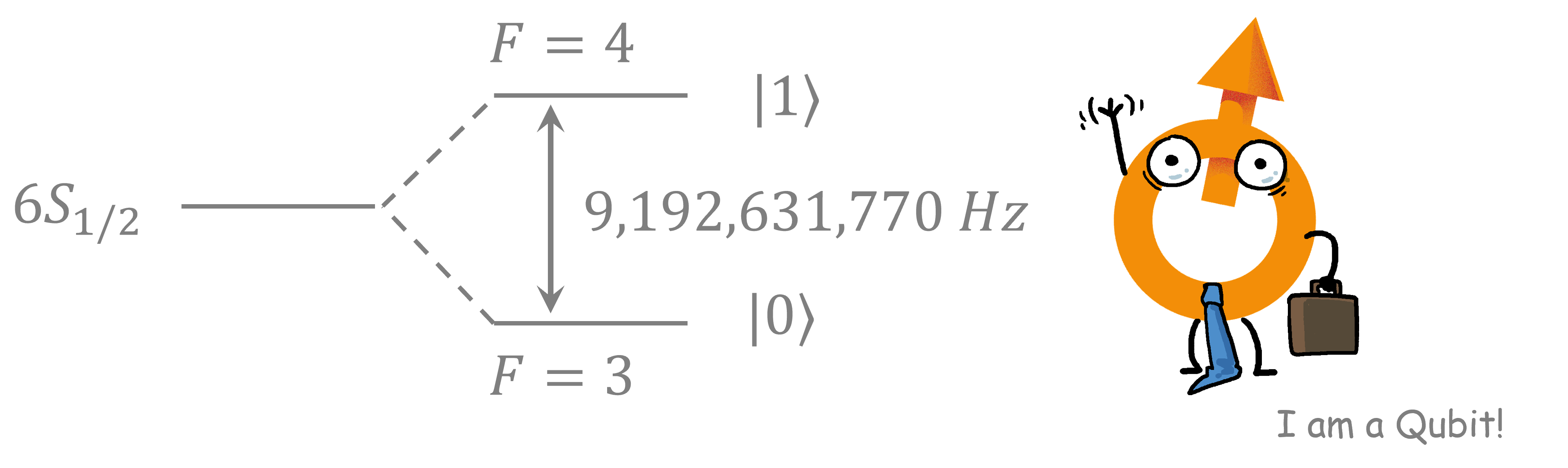In this lecture we shall focus on the latter approach and discuss, how quantum technology, can help improve the precision of phase measurements dramatically. We start by noting that the system in question is essentially a two-level system, or, in terms of Quantum Technology a QuBit. This is a very helpful observation as it allows us to draw on a set of theoretical understanding that we can exploit. The second is that we can now translate the phase measurement process on a quantum computer and do life-experiments in the classroom.

## Single QuBit based Quantum Phase Measurement¶

We first start by understaing the way phases are measured. This, of course, required interferometry, so we first have to create a superposition state, using the $\hat{H}$ operator from a computational basis state $|0\rangle$. Then we apply the phase shift using a phase gate $\hat{U}(\phi)=|0\rangle\langle0|+e^{i\phi}|1\rangle\langle1|$. Then we rotate by $\pi/2$ around the X-axis using the $\hat{RX}$-gate. Then we apply another $\hat{H}$-gate.

We use QISKIT to implement the interferometer operation:

In :
#This is just a few packages which we have to import first
from qiskit import QuantumRegister, ClassicalRegister, QuantumCircuit, Aer
from qiskit.visualization import plot_bloch_multivector
from numpy import pi
import numpy as np
import matplotlib.pylab as plt
sim = Aer.get_backend('aer_simulator')

<frozen importlib._bootstrap>:219: RuntimeWarning: scipy._lib.messagestream.MessageStream size changed, may indicate binary incompatibility. Expected 56 from C header, got 64 from PyObject

In :
phi = 0.1*np.pi
qc = QuantumCircuit(5)
#the 0-qubit remains empty
#the 1-qubit: mixing with the H-gate
qc.h(1)

#the 2-qubit:apply phase and rotate around along the equator
qc.h(2)
qc.p(phi, 2)

#the 3-qubit:rotate around x to get onto the zeor-meridian
qc.h(3)
qc.p(phi, 3)
qc.rx(pi/2,3)

#the 4-qubit: attempt to unmix with second H-gate. End up on a latitude proportial to phi
qc.h(4)
qc.p(phi, 4)
qc.rx(pi/2,4)
qc.h(4)

#we want to see the statevectors
qc.save_statevector()

#draw the circuit
qc.draw()

Out: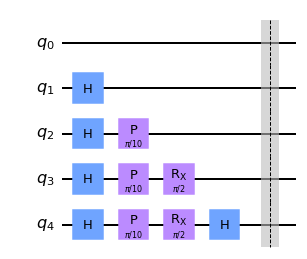In :
#draw the bloch-sphere
sim = Aer.get_backend('aer_simulator')
result = sim.run(qc).result();
state = result.get_statevector()
plot_bloch_multivector(state)


Out: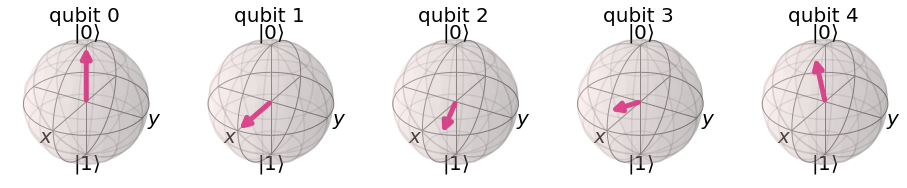Using this operation we have converted the phas $\phi$ into a measurable probability of the $|1\ket$-state, which you can see from the fact that the Block vector no longer points to the north pole. Let's do a bit of mathematics to find a quantitaive relation. The probability of measuring the Qubit in state $|0\rangle$ is $p_0=(1+\cos\phi)/2$ and in state $|1\rangle$ is $p_0=(1-\cos\phi)/2$.

We can thus extract the phase by measuring the parity $P=p_0-p_1$. The appropriate operator is given by $\hat{P}=|0\rangle\langle0|- |1\rangle\langle1|$ and the expectation value $\langle\hat{P}\rangle=\cos\phi$. It is also noteworthy that $\hat{P}^2=1$ and thus we find the variance $\Delta P^2 = \langle\hat{P}^2\rangle- \langle\hat{P}\rangle^2=\sin^2\phi$.

Experimentally we do not have access to the expectation value directly. The thing we can do is to use a (sufficiently large) sample $N$ of identical Qubits and measure those, recording the number of time $N_0$ that the Qubit collapsed into the $|0\rangle$ state and the number of times $N_1$, where it collapsed into the $|1\rangle$ state. From this we can construct the sample mean $\bar{P}=\frac{1}{N}(N_0-N_1)$ as an unbiased estimator for the expectation value $\langle\hat{P}\rangle$. From rather general assumptions on the probability distribution we can assume the this sample mean has a variance of $\Delta \bar{P}^2=\Delta P^2/N$. The mean error $\Delta \bar{P}$ is simply the square root of teh variance, which is $\Delta \bar{P}=\Delta P/\sqrt{N}$.

It was our task to measure $\phi$ with as little error $\Delta\phi$ as possible. Given that $\phi=\arccos{p}$ we find that the experimental error (noise level) $\Delta\bar{\phi}=\frac{1}{\sqrt{1-\bar{P}}}\Delta \bar{P}=\frac{1}{\sqrt{1-\cos{}^2\phi}}sin{}\phi\frac{1}{\sqrt{N}}=\frac{1}{\sqrt{N}}$.

This is a profound finding. It means that any measurement of the phase $\phi$ is fundamentally noisy with a fixed noise level, that scales with an inverse square with the number of QuBits that we can independently measure to fix a measurement. We need to incease the number of Qubits by 100 if we want to reduce the noise 10-fold. This also means that any clock will give a fundamental uncertainty in the timing on the order of $1\:\mathrm{rad}$ of a complete clock cycle divided by the square root for the number of identical resonators, which work in the clock.

### Task 1: Time Averaging of Independent QuBits¶

1. Create a quantum circuit that measures $\bar{\phi}$ for a given value of $N$ and $\phi$. Show the circuit.
2. Run the script as a function of $\phi\in(0,\pi)$ for a given number of $N=100,400,1600$ independent QuBits. Since you know the real value of $\phi$ you can plot the error $\Delta\phi=\bar{\phi}-\phi$ for every value of $N$
3. Plot the experimental error distribution for every value of $N$
4. Show for each $N$ that your estimator is unbiased (e.g. the mean error is zero) and that its standard devation follow the relation derived above.

We start by creating a circuit for the phase/parity measurement, as presented above. The specific phase $\phi$ goes in as an input parameter

In :
def phase_detection_single(phi):
qreg_q = QuantumRegister(1, 'q')
qc = QuantumCircuit(qreg_q)
qc.h(qreg_q)
qc.p(phi, qreg_q)
qc.rx(pi/2,qreg_q)
qc.h(qreg_q)
qc.measure_all();
return qc


Then we show one specific circuit for an arbitrary angle of $\phi=0.2\pi$ as an example.

In :
qc = phase_detection_single(0.2*np.pi)
qc.draw()

Out:Now we create a script that creates and runs the quantum script for a series of $\phi$-values, ranging from $0$ to $\pi$ (everything else will give problems, due to the arccos-ambiguity and I am too lazy for this). From teh results it calculates the sample estimated parity and also the sample-estimated phase estimated. The function return the series of true phases $\phi$, the series of sample estimated $\bar{P}$ and the series of sample estimated $\bar{\phi}$. The function takes the number for $\phi$ and the number of Qubits $N$ to be measured as inputs.

In :
def phase_detection_single_experiment(num_phi, N=1024):
sim = Aer.get_backend('aer_simulator')
parity = np.zeros([num_phi])
phases = np.zeros([num_phi])
phis = np.linspace(0,np.pi,num_phi)
for k, phi in enumerate(phis):
circuit = phase_detection_single(phi)
result = sim.run(circuit, shots=N).result();
counts = result.get_counts()
for key, val in counts.items():
key_ = key.replace(" ", "")
p = int(key_,2)
if p == 0:
parity[k] = parity[k]+val
else:
parity[k] = parity[k]-val

parity[k] = parity[k]/N
phis_est = np.arccos(parity);
return phis, parity, phis_est


This function is used to plot the results. It plots three curves

1. A plot of the sample estimated $\bar{P}$ values as a function of the true phase $\phi$ with an overlay of the true expectation value $\langle\hat{P}\rangle$.
2. A plot of the phase estimation error $\bar{\phi}-\phi$ as a function of the true phase $\phi$. This should be a constant zero-centered line.
3. A histogram of all phase estimation errors $\bar{\phi}-\phi$ over (should be independent of $\phi$, thus we can use this as an unbiased sampling method for the distribution of the errors). Together with the average error (should be $0$ because the estimator $\bar{\phi}$ is unbiased and with a standard deviation, which should be $\frac{1}{\sqrt{N}}$ as per the disussion above.

The function takes the result from phase_detection_single_experiment() as an input and return the list of phase estimation errors $\bar{\phi}-\phi$ as well as this lists mean and this lists standard devation (as displayed in the histogram).

In :
def phase_detection_single_experiment_plot(phis, parity, phis_est, N):
err = phis_est-phis
meanerr = np.sum(err)/num_phi
stddeverr = np.sqrt(np.sum((err-meanerr)**2)/(num_phi-1))

plt.figure(figsize=(12,8), facecolor='white')
plt.rcParams.update({'font.size': 22})
plt.plot(phis, parity,'bo')
plt.plot(phis, np.cos(phis),'b')
plt.title('N=' + str(N))
plt.xlabel('Phase $\phi$ [rad]')
plt.ylabel('Parity')
plt.xlim(0, np.pi)
plt.grid(which='major',axis='both')

plt.figure(figsize=(12,8), facecolor='white')
plt.rcParams.update({'font.size': 22})
plt.plot(phis, err,'bo')
plt.title('N=' + str(N))
plt.xlabel('Phase $\phi$ [rad]')
plt.ylabel('Phase Measurement Error')
plt.xlim(0, np.pi)
plt.grid(which='major',axis='both')

plt.figure(figsize=(12,8), facecolor='white')
plt.rcParams.update({'font.size': 22})
plt.hist(err)
plt.title('$N$=' + str(N) + ' MeanErr=' + f"{meanerr:{4}.{2}}" + ' StdDevErr=' + f"{stddeverr:{4}.{2}}")
plt.xlabel('Phase Measurement Error')
plt.ylabel('No. of Occurences')
plt.grid(which='major',axis='both')

return err, meanerr, stddeverr


Now we run the experiment with $N=100,400,1600$ as stated above

In :
num_phi = 100
N = 100
phis, parity, phis_est = phase_detection_single_experiment(num_phi, N)
err, meanerr, stddeverr = phase_detection_single_experiment_plot(phis, parity, phis_est, N)

N = 400
phis, parity, phis_est = phase_detection_single_experiment(num_phi, N)
err, meanerr, stddeverr = phase_detection_single_experiment_plot(phis, parity, phis_est, N)

N = 1600
phis, parity, phis_est = phase_detection_single_experiment(num_phi, N)
err, meanerr, stddeverr = phase_detection_single_experiment_plot(phis, parity, phis_est, N)